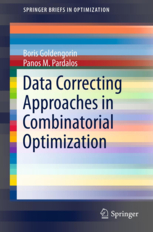• A
• A
• A
• ABC
• ABC
• ABC
• А
• А
• А
• А
• А
Regular version of the site

## Data Correcting Approaches in Combinatorial Optimization

NY: Springer, 2012.
Goldengorin B. I., Pardalos P. M.

Data Correcting Algorithms in Combinatorial Optimization focuses on algorithmic applications of the well known polynomially solvable special cases of computationally intractable problems. The purpose of this text is to design practically efficient algorithms for solving wide classes of combinatorial optimization problems. Researches, students and engineers will benefit from new bounds and branching rules in development efficient branch-and-bound type computational algorithms. This book examines applications for solving the Traveling Salesman Problem and its variations, Maximum Weight Independent Set Problem, Different Classes of Allocation and Cluster Analysis as well as some classes of Scheduling Problems. Data Correcting Algorithms in Combinatorial Optimization introduces the data correcting approach to algorithms which provide an answer to the following questions: how to construct a bound to the original intractable problem and find which element of the corrected instance one should branch such that the total size of search tree will be minimized. The PC time needed for solving intractable problems will be adjusted with the requirements for solving real world problems.